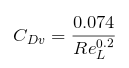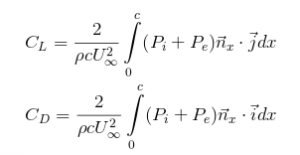# AIRFOIL SHAPE OPTIMIZATION 3 – Genetic Algorithm

This tutorial follows the tutorial about POD surrogate model on the airfoil shape optimization case. Thanks to a 3 design points Design of Experiment, we managed to generate a POD basis for the pressure field around this airfoil. Based on this POD basis, we are now able to predict the behavior of the pressure field around the airfoil on any new set of parameters (new airfoil shape) within the DOE bounds.

POD basis for pressure field around the airfoil

Thanks to this surrogate model (POD basis + interpolation method) we are now able to make fast design space exploration. Based on fast pressure field prediction, it is now possible to compute drag and lift coefficients at each cycle of the optimization process. Let’s see how to set up the optimization phase.

## Drag and Lift computation

The goal of the optimization phase will be to minimize the drag over lift ratio Cd/Cl. The lift can be directly computed from the pressure field, but the drag is divided between pressure drag and friction drag. As the flow around the airfoil stay attached, we will consider that the friction drag is not varying much. To compute it we will use the following model :With a Reynolds number of 3e6. The pressure-linked coefficients can then be computed thanks to the following formulas :Based on the 2D airfoil upper and lower coordinates (resp. airf2Du and airf2Dl) and associated pressure (resp. pAirfu and pAirfl) on each of the airfoil points (in the same order), you can compute the drag and lift coefficients.

The following table gathers the comparison between the OpenFOAM simulated values and the Scilab computation values of the coefficients for each DOE points. As we can see, the computation of pressure drag Cdp and lift Cl is good. Moreover, as assumed, the friction drag Cdv does not vary much. So it is relevant to make optimization only considering pressure drag.

## Cost function

We are now able to express in Scilab our optimization goal : Minimizing f = Cd/Cl. Function f is called cost function. But this function is always hard to set.

• Genetic algortihm cost function

In this case, you only have to define the function itself. Be careful though, because using Scilab min function will lead you to negative values of the cost function when you want it to be as small as possible. You can try to minimize the absolute value of the ratio Cd/Cl, but in this case (non-constrained optimization), you allow the lift coefficient to be negative, and that is not optimal. You can for example set the following cost function.

Setting one set of parameters x, you can compute your new pressure field around the airfoil leveraging the POD basis and interpolation (interp4NewFoil function). Based on that, it is possible to compute drag and lift coefficients (LaDComput function) around the new airfoil (newAirfoil function) and return the value of the cost function f.

This case is a bit more complex than the previous one. Actually, for gradient descent method you have to implement your own cost function gradient. So, if you want to keep it simple, avoid to much non-continuous cost function. Anyway, those algorithm only gives you local optimum. So you will have to try different initial conditions to find a good optimum. Hopefully, those algorithm are running quite fast.

This case obviously need the evaluation of more pressure field (1 basic case + 6 gradient cases) in order to compute the 3-components gradient corresponding, one component for one parameter.

This case is easy to set. Just define your parameters lower and upper bounds (resp. binf and bsup), set an initial value for the parameter x0 and then use the optim function defining your cost function. Finally you get your minimum cost function fopt and the associated set of parameters xopt.

As said above, this method provides you with local optimum following the implemented gradient. If you want to be sure of your optimum, you will have to run your optimization for different initial conditions x0 that correspond to different gradient directions.

## Genetic Algorithm optimization

Compared to the gradient descent method, this method provides you with an approximation of the global optimum. Those methods are metaheuristic directly inspired by the process of natural selection. Basically, you start with an initial population (of set of parameters x) generated random within the bounds defined in myParam, and of size PopSize. This population will be affected at each generation (cycle) by “natural processes”such as selection, cross-over and mutation with a given probability (probaMut and probaCross for resp. mutation and cross-over), changing the population within the parameters bounds. Those “natural processes” are functions that you can change is you want., and will be applied for a given number of cycle (NbGen).

The optim_ga function will then give you the optimal population pop_opt of set of parameters that minimize the cost function.

## Conclusion

This 3 parts tutorial is now finished. The global process of airfoil shape optimization gave the following result : xopt = [0.05 0.02 0.02].

Simulated velocity field and pressure coefficients for new optimal airfoil (vs NACA 0012)

This airfoil correspond to the first point of the DOE. This result is not really surprising though, considering the low flow Mach number. But the previous result is not as interesting as the complete process set for this case. Now that you know how to make the set the same analysis, it’s your turn to generate better surrogates models for your design optimization study.